## Objective Questions from Circuit Theory:

 A balanced RYB-sequence,Y-connected(Star Connected) source with VRN=100 volts is connected to a Δ-connected (Delta connected) balanced load of (8+j6) ohms per phase.Then the phase current and line current values respectively,are[IES2010]
(a) 10A;30A
(b) 10√3A;30A
(c) 10A;10A
(d) 10√3A;10√3A

(e) None of the above

 Assuming both the voltage sources are in phase, the value of R for which maximum power is transferred from circuit A to circuit B is [GATE2012](a) 0.8Ω
(b) 1.4Ω
(c) 2Ω
(d) 2.8Ω
(e) None of the above

 The voltage gain Av of the circuit shown below is [GATE2012]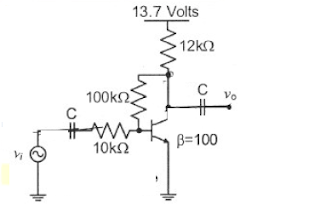(a)  |Av200
(b)  |Av|100
(c)  |Av|20
(d)  |Av|10
(e) None of the above

 If VA-VB=6V,  then VC-VD is [GATE2012]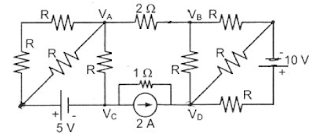(a)  -5V
(b) 2V
(c) 3V
(d) 6V
(e) None of the above

Solution Hint:
As Va-Vb =6  => I = V/R = 6/2 = 3A
entering current = leaving current [ KCL]
so current from Vd to Vc is same  as 3A
convert the current source to voltage source
V = IR =2 x 1 = 2V

Vd = Vc + 2V + (3x1)V = Vc + 5V
Vc-Vd = -5V

The circuit shown is a [GATE2012](a) Low pass filter with f3db=[1/(R1+R2)C] rad/s
(b) High pass filter with f3db=[1/(R1C)] rad/s
(c) Low pass filter with f3db=[1/(R1C)] rad/s
(d) High pass filter with f3db=[1/(R1+R2)C] rad/s
(e) None of the above

 In the circuit shown, an ideal switch S operated at 100kHz with a duty ratio or 50%. Given thatΔic 1.6A peak-to-peak and I0 is 5A dc, the peak current in S is [GATE2012](a) 6.6A
(b) 5.0A
(c) 5.8A
(d) 4.2A
(e) None of the above

Solution Hint:
Δic = 1.6A peak-to-peak
The positive peak  = Δic /2
The current flows through the switch has a peak of =  Io +  Δic /2
For your information...The current waveform is like this
The lowest point will be 5A....highest point will be 5+ 0.8

 The i-v characteristics of the diode in the circuit given below are
i={ [ V- 0.7 /500]A,   V > or = 0.7 V

0A,   V< 0.7 V

The current in the circuit is [GATE 2012]
(a)  10 mA
(b)  9.3 mA
(c)  6.67 mA
(d)  6.2 mA
(e) None of the above

Solution Hint:
we have to find v and then apply in ct. equ...
KVL...10 = 1K * i + v
10 = 1000i +v = 1000(v-0.7/500) + v
solve it ....v= 0.8...

 In the following figure, C1 and C2 are ideal capacitors. C1 has been charged to 12V before the ideal switch S is closed at t=0. The current i(t) for all t is  [GATE 2012]
(a) Zero
(b) A step function
(c) An exponentially decaying function
(d) An impulse function
(e) None of the above

Solution Hint:
If it is RC ckt...ie any Resistance in series with capacitor, it will be an exponentially decaying function....here no resistance...so charge instantly....ie impulse function...

 The impedance looking into nodes 1 and 2 in the given circuit is [GATE 2012]
(a) 50 Ω
(b) 100 Ω
(c) 5 KΩ
(d) 10.1 KΩ
(e) None of the above

 In the circuit given below,the current through the inductor is [GATE 2012]

(a)  (2/1+j)A
(b)  (-1/1+j)A
(c)  (1/1+j)A
(d)  0A
(e) None of the above

Solution Hint: consider top half of the circuit
R & L are in parallel with ct. source....other end connected to low potential...ie GND... convert ct. to V source...
4:40 PM

1.Sir,this blog is so much helpful..and one small request sir,
please provide solutions also for the questions sir.
Thank u

1.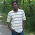Thanks for your comments...ans hint is added...For future post we will give solutions hint...

2.For previously published posts, please let us know the question no, we will add the soultion hint for that answer.

2.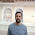thanks sir for your valuable efforts .......
It may be great if you add next post link at end of every post .......
Make playlist like youtube

thanks again

3.sir please provide objective type for power quality engineering also and also give explanations

4.really it is very useful to all students thank u sir

5.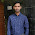Answer is C for Question No. 10

6.the question no 10 answer is C